Radiative Cooling and Precipitation Change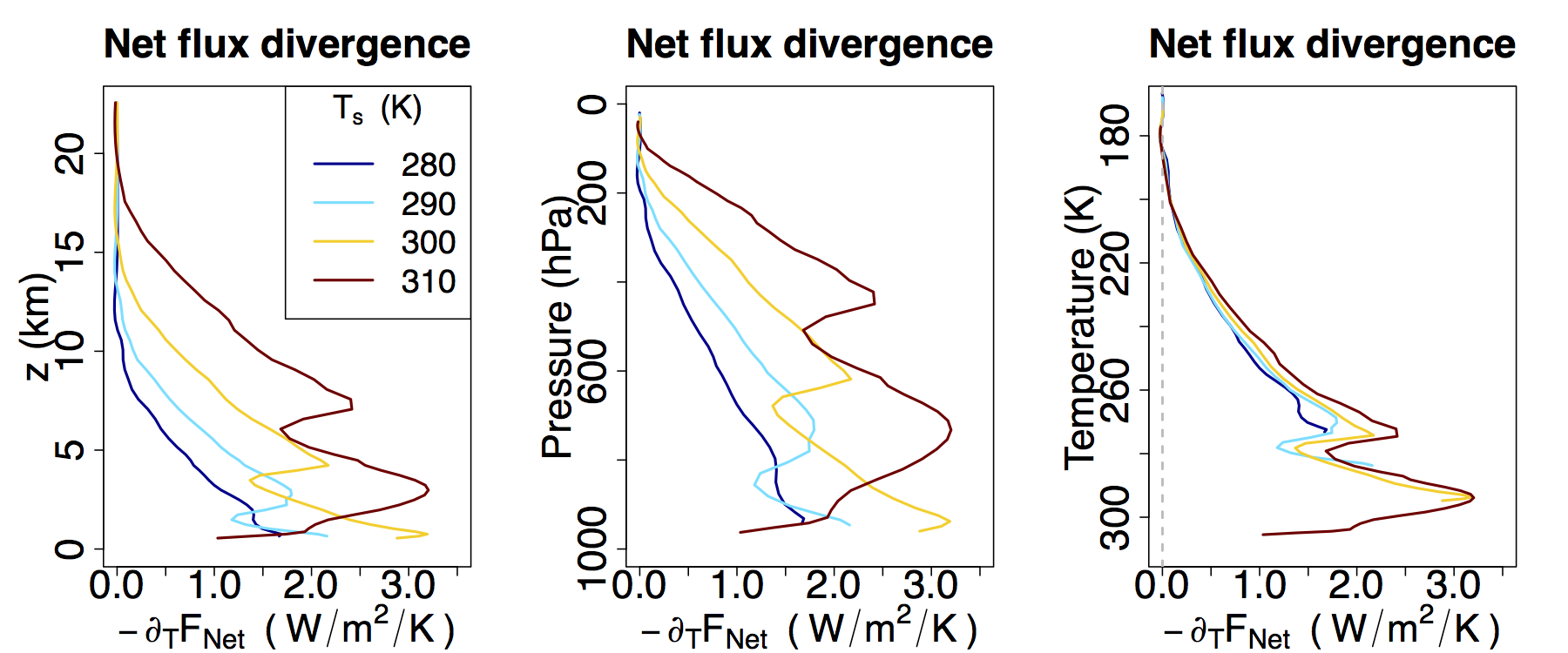Net radiative flux divergences from cloud-resolving RCE simulations at various surface temperatures Ts. Divergences are computed with respect to temperature as a vertical coordinate, but are then plotted as functions of z, p, and T. When plotted in T coordinates the divergence profiles collapse onto a common curve which extend downwards with increasing Ts.

Global mean precipitation is known to roughly balance global mean radiative cooling, and in simulations both increase with surface warming at a rate of roughly 2% per degree C. The origin of this value is not well understood, however. We explain this value by leveraging a novel invariance which radiative cooling profiles exhibit when calculated using temperature as a vertical coordinate (figure right). This invariance leads to a simple picture in which column-integrated radiative cooling is governed primarily by the depth of the troposphere, when measured in temperature coordinates. For more, see Jeevanjee and Romps (2018). (SI can be found here, and an alternate description of this work here).

Effective Buoyancy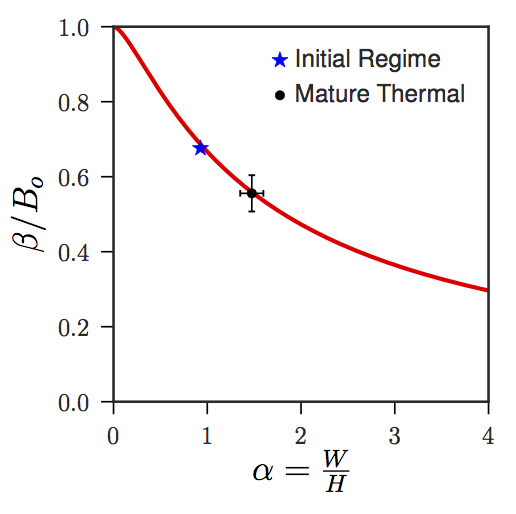Effective buoyancy β normalized by Archimedean buoyancy B0, as a function of parcel aspect ratio α (Width/Height). Red line is the theoretical curve, whereas the points are diagnosed from the spherical, laminar regime (blue star) and ellipsoidal, turbulent regime (black circle) of a DNS simulation of a single convecting parcel.

The 'effective buoyancy' of an accelerating parcel is its Archimedean buoyancy B minus an offset, due to the parcel having to push some of the environmental fluid out of its way. This offset grows with parcel aspect ratio, so that wider parcels (at fixed height) accelerate less. Through a novel correspondence with the equations of magnetostatics, we find exact analytical expressions for this offset for idealized parcels with uniform B, and show that these expressions also apply to turbulent, heterogenous parcels (figure left). These expressions describe the `virtual mass' effect for fluid parcels, as well as the compensating subsidence in their environment. See Tarshish et al. (2018) for complete details.

An earlier approach to some of these questions, which emphasizes how this offset of B is enhanced when parcels are near the surface, can be found in Jeevanjee and Romps (2016). An application of these ideas to understanding how simulated convection depends on model grid spacing is given in "Vertical Velocity in the Gray Zone", Jeevanjee (2017), discussed below.

Vertical Velocity in the Gray Zone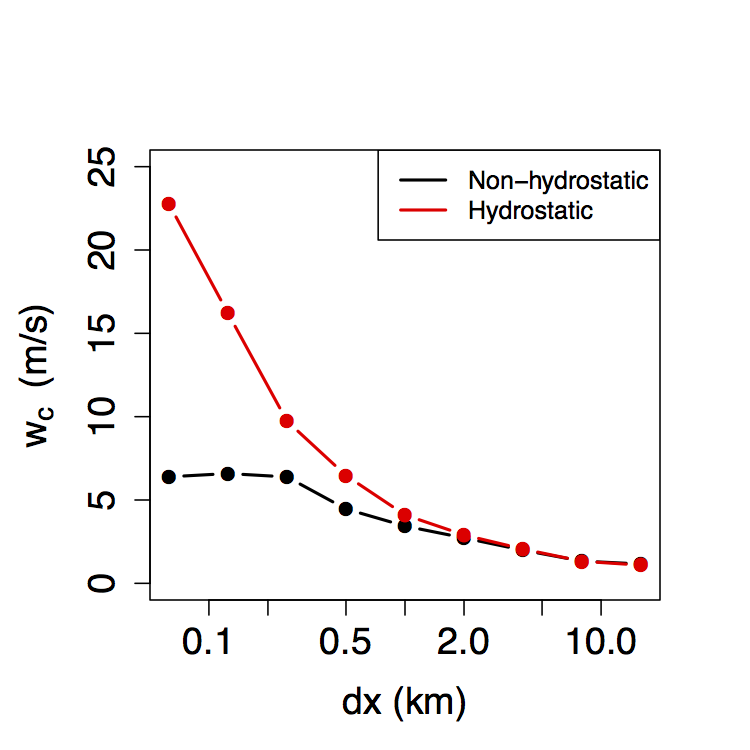Convective vertical velocities wc as simulated by FV3, as a function of horizontal resolution dx. Vertical velocities do not converge until dx < 250 m, and are over-estimated by a factor of 2 - 3 at these resolutions when the hydrostatic approximation is employed.

Increasing computer power allows global atmospheric models to be run in a `gray zone' of horizontal resolution which permits but does not fully resolve convection. It is unclear where this gray zone ends, however, and how it is affected by the oft-employed hydrostatic approximation. We address these questions using GFDL's flagship FV3 dynamical core, running simulations across the gray zone both with and without the hydrostatic approximation. We find that horizontal resolutions of approximately 100 m are required for convective vertical velocities wc to converge, and that the hydrostatic approximation over-estimates wc at these resolutions by a factor of 2 - 3 (see figure right). These behaviors of wc can be described by simple analytical formulae, which also map out how wc behaves throughout the gray zone.

See Jeevanjee (2017) for details, and here for an animation of cloud-resolving FV3. During the course of this study we also found a striking dependence of the modeled convection on the explicit damping used to dissipate grid-scale noise. This was investigated further in Anber et al. (2018).

Climate Model Hierarchies

Inspired by the WCRP's recent Model Hierarchies Workshop, we attempted to survey and synthesize some of the current thinking on climate model hierarchies. We give a few formal descriptions of the hierarchy (see figure below), and survey its various uses. We also discuss some of the pitfalls of contemporary climate modeling, and to what extent the `elegance' advocated for by Held (2005) has been used to address them. See Jeevanjee et. al. (2017) for more.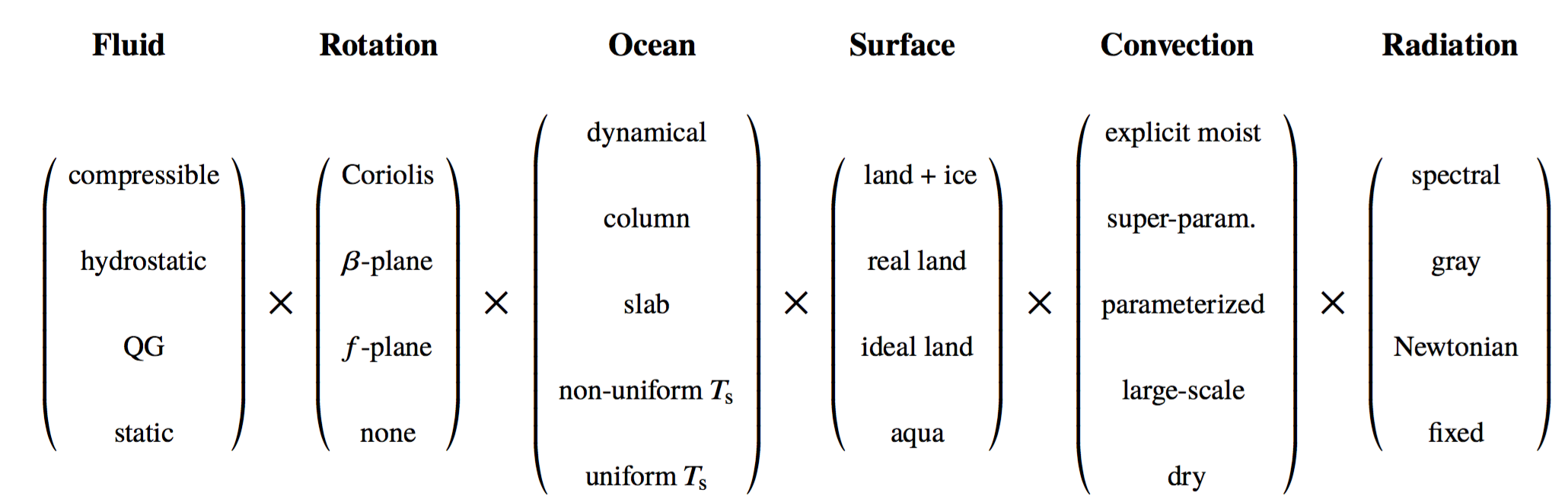The climate model `hierarchy' can be thought of as a cartesian product space of individually hierarchical axes, roughly corresponding to model components. Both the list of axes and the list of points within a given axis are chosen to be illustrative, rather than definitive.

Effective Buoyancy, Inertial Pressure, and Convective Triggering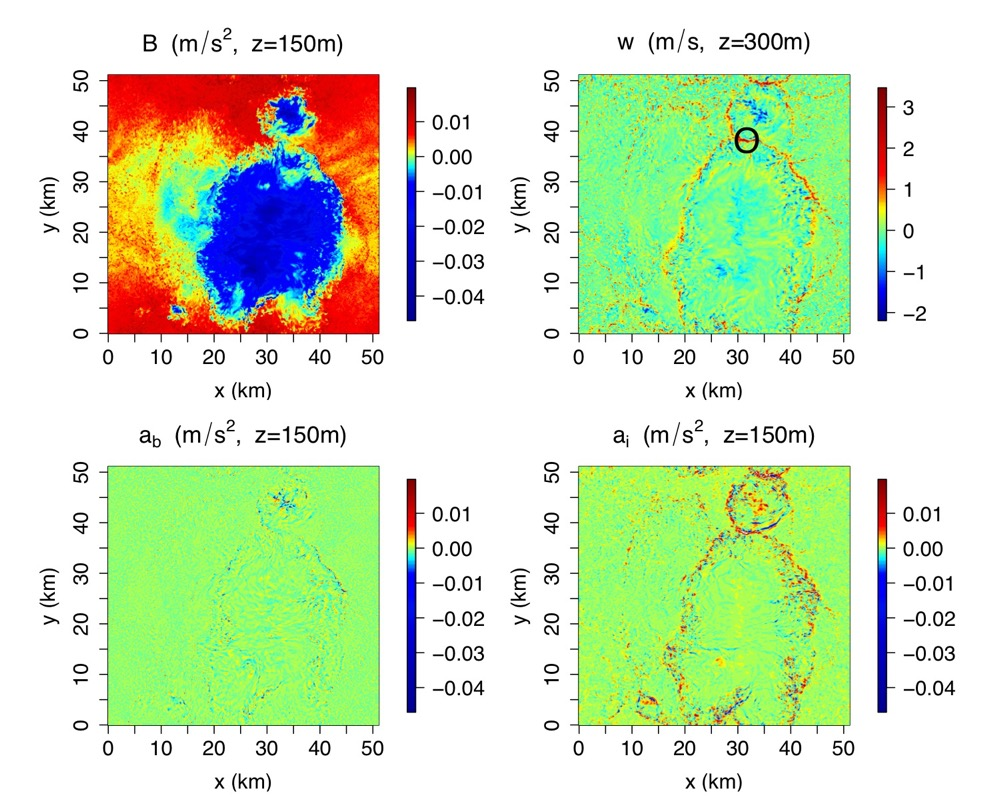Clockwise from upper left: snapshots of near-surface Archimedean buoyancy B, vertical velocity w, inertial acceleration ai, and effective buoyancy ab in a simulation of tropical deep convection.

The other force besides effective buoyancy acting on convecting parcels is the inertial (or dynamic) pressure force. In tropical deep convection, most new convection is generated on the edges of 'cold pools' of air produced by evaporation of rain from existing convection. (A large cold pool is visible in the B field in the figure to the right, and the triggered convection along its edge is visible in the w field). It had long been thought that inertial pressure was responsible for this triggering, but the results of Tompkins (2001) suggested that effective buoyancy might also contribute. We helped settle this question by numerically solving the Poisson equations for both effective buoyancy and inertial acceleration in a simulation of tropical convection, showing that indeed the inertial acceleration dominates (bottom row of figure).

See Jeevanjee and Romps (2015) for details.

Convective Self-aggregationSnapshots of near-surface specific humidity from simulations of various domain sizes, with cold pools "turned off". In this case aggregation is possible at all domain sizes (L is the width of each domain in km).

Simulations of tropical convection can spontaneously develop large-scale circulations even in the absence of any large-scale forcing, in a phenomenon known as "self-aggregation". This large-scale circulation partitions the atmosphere into moist and dry regions, as can be seen in the individual panels to the left. Such self-aggregation had previously only been seen in simulations with a domain larger than ~ 300 km on a side, but by disabling cold pools in our simulation, we obtained aggregation at all domain sizes (see figure).

See Jeevanjee and Romps (2013) for details.